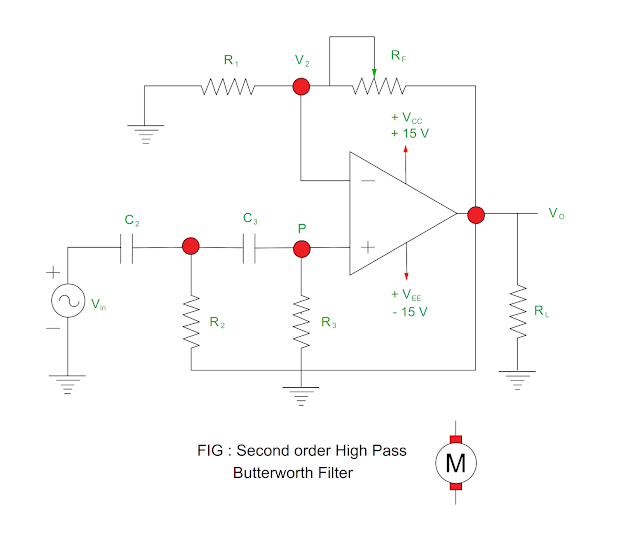## 24/07/2021

### High Pass Butterworth Filter

In this article, details study of first order high pass Butterworth filter, its frequency response, voltage gain, and second order high pass Butterworth filter is given.

# First order High Pass Butterworth Filter

• The high pass filter is obtained by changing frequency determining component – resistors and capacitors in the low pass filter.
• The first order high pass filter is obtained by interchanging R and C in the first order low pass filter.
• Figure shows first order high pass Butterworth filter.

## Lower cut off frequency

• It is frequency at which gain of the amplifier reduces from maximum to 70.7% of its maximum value.
• It is from frequency response graph, one can say that lower cut off frequency is determining parameter for stop band frequencies and pass band frequencies.

Impedance of the capacitor = – jXC

= – j ( 1 / ωC )

= – j / 2πfC

= 1 / j2πfC

Input voltage at point P according to voltage divider rule

VP = Vin { R / ( R – jXC ) }

= Vin { R / ( R + 1 / j2πfC  ) }

= Vin { R / (( j2πfRC + 1) / j2πfC  ) }

= Vin { j2πfRC / ( 1 + j2πfRC ) }

Let us consider that

Lower cut off frequency fL = 1 / 2πRC

Divide numerator and denominator by 1 /2πRC

= Vin { ( jf ) / ( 1 / 2πRC ) +( j2πfRC / 2πRC )

= Vin { jf / ( 1 / fL + jf ) }

= Vin { jf / ( 1 +jfL f ) / fL }

= Vin { jf fL / ( 1 + jfL f ) }

Divide numerator and denominator by fL2

= Vin { j( f / fL ) / ( 1 + j( f / fL )  }

Output voltage Vo = AF VP

Where AF = gain of the OP – Amp

= ( 1 + RF / R1 )

Output voltage Vo = AF VP

= ( 1 + RF / R1 ) { j( f / fL ) / ( 1 + j( f / fL ) } Vin

Voltage Gain: Vo / Vin = ( 1 + RF / R1 ) { j( f / fL ) / ( 1 + j( f / fL ) }

Magnitude of Voltage Gain: ( 1 + RF / R1 ) { ( f / fL ) / ( 1 + √ ( f / fL )2 }

= AF{ ( f / fL ) / ( 1 + √ ( f / fL )2 }

## Frequency response of high pass Butterworth filter

• When the frequency decrease that of lower cut off frequency, the high pass Butterworth filter rolls at – 20 dB / dec.
• The voltage gain reduced to 0.707AF at lower cut off frequency.
• The voltage gain becomes constant when frequency increases beyond lower cut off frequency.

 Frequency Voltage gain Low frequency < AF Lower cut off frequency = 0.707AF Greater than lower cut off frequency Constant

## Second order High Pass Butterworth Filter

• The second order high pass Butterworth filter can be obtained by interchanging frequency determining components R & C in the second order low pass filter.
• The second order high pass Butterworth filter is shown in the Figure.Magnitude of Voltage Gain: AF / √ { 1 + ( fL / f )2 }

Where

f = Input frequency

fL = Low cut off frequency

=  1 / 2π √ ( R) ( R) ( C) ( C)

AF = Pass band gain

You may also like :

Types of Filter Circuit

What is Mobility?

Power & Energy

#### 1 comment:

1.I liked your work and the way in which you have shared this article here about testing and tagging adelaide. It is a beneficial and helpful article for us. Thanks for sharing an article like this.